# An Emergency Management System for Government Data Security Based on Artificial Intelligence

An Emergency Management System for Government Data Security Based on Artificial Intelligence
State Information Center, Beijing 100045, China

Corresponding Author Email:
lhn@cegn.gov.cn
Page:
207-213
|
DOI:
https://doi.org/10.18280/isi.250208
10 November 2019
|
Accepted:
6 February 2020
|
Published:
15 May 2020
| Citation

OPEN ACCESS

Abstract:

This paper designs a novel emergency management system for government data security based on artificial intelligence (AI). Firstly, a novel concept was put forward government data resilience chain (GDRC) based on the AI technology. Next, several AI techniques were designed to ensure the security of government data on data sharing and exchange platforms, including intelligent government data security monitoring technology, artificial neural network (ANN) and fuzzy identification technology, and firewall security technology based fuzzy clustering. On this basis, an emergency management system was established for government data security, and verified through simulation of fault tolerance of multi-agent formation. The results show that the GDRC helps solve emergency management problems in the sharing and exchange of government data; the proposed system realized deep learning (DL), and real-time updates of dynamic features of multiple agents. The research results provide effective decision supports for improving the fault tolerance of multi-agent system.

Keywords:

government data resilience chain (GDRC), emergency management, multi-agent formation, fault tolerance

1. Introduction

In recent years, many countries have established data sharing and exchange platforms, which meet the data demand of the government and the public. However, the government data are not sufficiently open or standardized . There is a severe lacking of disclosure standards and regulatory mechanisms for data on national emergency management. To make matters worse, the government’s preparation and utilization of open data have not been verified, in the light of the key issues in emergency management.

Artificial intelligence (AI) offers a possible solution to the above problem. Currently, the AI techniques combine the merits of deep learning (DL) algorithms, and the information processing strategies of big data [2-6]. Machine learning (ML), a subset of the DL, makes full use of the superior computing accuracy and data processing capabilities of machines. Many scholars have explored emergency management, using ML methods for information flow, edge computing, secure delegation of private data, etc. [7-10].

This paper attempts to ensure the security of data sharing and exchange platforms in the area of emergency management. For this purpose, a novel concept called government data resilience chain (GDRC) was put forward based on the AI techniques for government data security. Based on the GDRC, an emergency management system was established to ensure the security of government data. The application in multi-agent formation proves that our system guarantees the robustness of the business at the location of government data, and safeguards the sustainable and smooth operation of important business platforms.

2. Security Model

Drawing on traditional data security models, this section constructs an AI-based security model for government data in emergency management systems. The purpose of the model is to enhance the reliability of the decision supports to emergency response . The mainstay of the security model is the GDRC, which consists of four key aspects: continuous monitoring, efficient response, fast recovery, and repeated verification. The structure of the GDRC-centered security model is illustrated in Figure 1 below.

Specifically, continuous monitoring, the core of the GDRC, fully utilizes DL and other AI techniques to extract the features of various existing or potential security threats, classifies the extracted features, and combines the classification results into a feature library. Efficient response refers to the efficient research and actions in response to the contents of continuous monitoring. Fast recovery protects and restores government data based on the responses. Repeated verification relies on techniques, personnel, and processes to verify the latest system state on a continuous basis, offering positive feedbacks for continuous monitoring.

## 2.1.png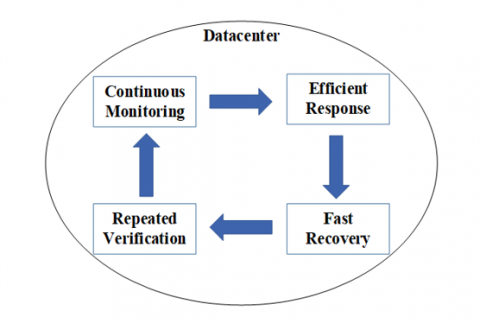Figure 1. The GDRC-centered security model

3. AI-Based Security Techniques

In the area of government data security, the main application value of the AI lies in its strong ability to gather information resources, analyze and process fuzzy information, make effective learning and reasoning, as well as cooperate with multiple agents. Thanks to these advantages, the AI boasts strong applicable value in protecting the security of government data. This section brief introduces the AI techniques adopted to ensure government data security.

3.1 Government data security monitoring technology

Based on AI techniques like the DL and big data, government departments at all levels can establish big data security centers to collect, organize, and analyze various types of security events, and then build a data security detection and response platform covering such dimensions as region, industry, and type. The multi-dimensional platform will provide each datacenter with network security services from the cloud. With the aid of AI techniques, the government departments will be able to respond quickly to unexcepted security incidents, e.g. fast-tracking incident analysis, alert release, and information processing. The security strategy of the multi-dimensional platform will be updated synchronously, creating a complete and efficient data security system.

3.2 Artificial neural network (ANN) and fuzzy recognition technology

The ANN is a highly self-adaptive network capable of identifying noises or distortions. Mimicking the information interaction principle of the biological neural network, the ANN acquires the learning and comprehension abilities to quickly identify and store various information. Based on the ANN, a time series prediction model can be established to improve the detection efficiency of intruding viruses. In addition, the fuzzy recognition technology, also an AI technique, can be introduced to screen and pinpoint viruses rapidly in each datacenter, allowing the government to determine the type of viruses and adopt the correct security policies for the computer network.

3.3 Firewall security technology based on fuzzy clustering

The firewall security is an AI technology with advanced intelligence and decision-making capabilities. This technique helps to further enhance the robustness of network security. There are multiple sub-techniques of the firewall security, including but not limited to fuzzy clustering, packet filtering, and state monitoring. Among them, fuzzy clustering can predict unknown risks based on the current feature library, and detect the intruding viruses in an accurate manner. Packet filtering mainly performs nonlinear monitoring of data packets n the network layer, and integrates the destination address and source information.

4. System Design Based on Multi-Agent Formation

In the era of the big data, it is of great importance for government departments to ensure the security of data sharing and exchange platforms. From the perspective of multi-agent collaboration, this paper builds up an emergency management system for government data security. In this system, each agent represents a datacenter. Dividing the datacenters into masters and slaves, the following analysis only focuses on the agents of master datacenters.

For our problem, three agents are sufficiently representative, as the situation of additional agents can be determined by analogy. To ensure the security of datacenters, it is critical to find the formation of agents that maintain the fault tolerance and persistence of the emergency management system [12,13]. Here, the fault tolerance is only considered under the situation where only one connection of agent is cut off.

4.1 Preliminaries for graph persistence

The previous studies have shown that formation can fulfil various tasks in different ways, and the formation should be controlled by directed graph rather than undirected graph [14-17]. Hence, it is necessary to express the underlying rigid formations as an undirected graph.

Let $G=(V, E)$ be an undirected graph with $n$ points, $p=$ $\left(p_{1}, p_{2}, \ldots p_{n}\right) \in R^{2 n}$ as the composite vector, and $(G, p)$ be a pair of points. Note that $p_{i}(i \in\{1,2, \ldots, n\})$ is the coordinates of the i-th point on the plane. Further, a constant parameter $d_{i j}=\left\|p_{i}-p_{j}\right\|$ was introduced as the Euclidean distance between a pair of points $\left(p_{i}, p_{i}\right) .$ In addition, the rigidity matrix was defined as :

$\left\langle ({{p}_{i}}-{{p}_{j}}) , ({{p}_{i}}-{{p}_{j}}) \right\rangle =d_{ij}^{2} i,j\in \{1,2,\ldots ,n\}$     (1)

Assuming that the trajectory is smooth, the following can be derived from formula (1):

\begin{align} & 2\left\langle ({{p}_{i}}-{{p}_{j}}) ,(\overset{\centerdot }{\mathop{{{p}_{i}}}}\,-\overset{\centerdot }{\mathop{{{p}_{j}}}}\,) \right\rangle =0, \\ & i,j\in \{1,2,\ldots ,n\}, t\ge 0 \\ \end{align}      (2)

where, $\dot{p}_{i}$ is the velocity at point $p_{i} .$ The following homogenous function can be obtained by combining formula (2) at different points:

$R\overset{\centerdot }{\mathop{p}}\,=0$       (3)

where, $\dot{p}=\operatorname{column}\left\{\dot{p}_{1}, p_{2}, \ldots p_{n}\right\} ; R$ is a rigidity matrix with dimension $m \times 2 n[m=n(n-1) / 2]$ on the plane.

By another way of definition, the rigidity function can also be described as follows:

${{\overset{\wedge }{\mathop{g}}\,}_{G}}(p)={{[ ||p_{{{e}_{1}}}^{in}-p_{{{e}_{1}}}^{out}|{{|}^{2}},||p_{{{e}_{2}}}^{in}-p_{{{e}_{2}}}^{out}|{{|}^{2}},\ldots ||p_{{{e}_{m}}}^{in}-p_{{{e}_{m}}}^{out}|{{|}^{2}} ]}^{T}}$     (4)

where, the $i$ -th component $\left\|p_{e_{k}}^{i n}-p_{e_{k}}^{\text {out}}\right\|^{2}$ corresponds to the $\text { edge } e_{k} \operatorname{of} E\left(k \in\{1,2, \ldots, m\} E=\left\{e_{1}, e_{2}, \cdots e_{m}\right\}\right)$

Definition 4.1 [19, 20] A framework $(G, p)$ is infinitesimally rigid on the plane if $\operatorname{dim}\left(\operatorname{ker} J_{\hat{g}_{G}}(p)\right)=3, \text { or if }$

$\text{rank}{{J}_{{{\overset{\wedge }{\mathop{g}}\,}_{G}}}}(p)=2n-3$     (5)

where, $J_{\hat{g}_{G}}(p)$ is the Jacobian matrix of $\hat{g}_{G}(p)$ and takes the same form as the rigidity matrix $R$

Rigidity is the property of the undirected graph, and persistence is the corresponding property of the directed graph.

Lemma 4.1 For a directed graph with more than one point, it is minimally persistent if and only if the underlying undirected graph is minimally rigid, and of no point has more than two outgoing edges .

It is known that the number of the points in minimally persistent graph is always 2n-3, where n is the number of points.

4.2 Formation control laws

Figure 2 gives an example of the minimally persistent formation of three co-leaders (agents 4, 5, and 6). The three leaders in the cycle all have one degree of freedom (DOF). The DOF of followers (agents 1, 2, and 3) is zero. The followers are directly or indirectly controlled by the leaders through the distance constraint. Specifically, agent 1 is controlled by agents 4 and 6; agent 2 is controlled by agent 4 and follower 1.

## 2.2.png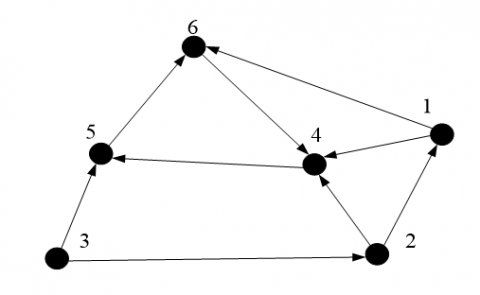Figure 2. A minimally persistent formation of three co-leaders

In this paper, the first-order kinematic model is adopted for every agent:

${{u}_{i}}=\overset{\centerdot }{\mathop{{{p}_{i}}}}\,$    (6)

Suppose the adjustment of leaders is so minor that the followers can catch up with the two neighboring leaders in due course. The formation motions were detailed as follows:

Before time 0, the agents are in the persistent statement that every distance and direction constraints are working, fulfilling the requirements on rigidity and constraint consistence. Then, one leader discovers the object, moves to it, and stops moving for a while. The other two leaders will quickly follow suit. After that, all followers will correct their positions based on the distance constraint with their neighboring leaders. Next, the first leader will move again. The above process finishes in a very short time, and will be repeated on and one. However, the control laws for leaders and followers are different, for the two types of agents follow diverse motion principles.

4.2.1 Control laws for leaders with fault tolerance

Suppose three leaders n-2, n-1, and n lead each other, and any of them responds to the formation assignment randomly. As shown in Figure 3, leaders 4, 5, and 6 are equivalent. It is assumed that leader 6 is the first to respond to the assignment. Thus, leaders 4 and 5 need to respond to leader 6. The dashed lines are specially designed spare lines (ineffective in normal motion) for the fault tolerance of the leaders in the cycle.

## 2.3.pngFigure 3. A minimally persistent formation of three co-leaders with fault tolerance

There are three different formations of co-leaders for fault tolerance:

(A) Disconnection at edge (6, 4)

First, it is assumed that the edge between leaders 6 and 4 is broken (Figure 4). As shown in Figure 5, when edge (6, 4) is cut off, leaders 4 and 5 still know the information from leader 6, and the added edge (4, 6) or (5, 4) does not take a part in the control laws.

## 2.4.png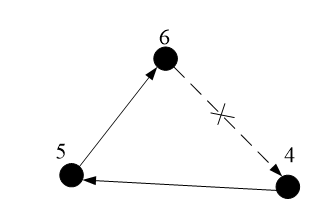Figure 4. Disconnection at edge (6, 4)

## 2.5.png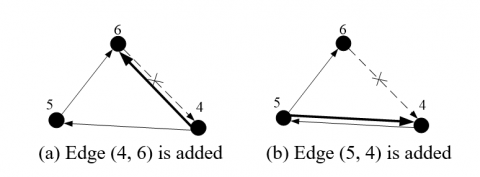Figure 5. Type A fault tolerance

Hence, the detailed control laws can be expressed as:

$\overset{\centerdot }{\mathop{{{p}_{n-2}}}}\,={{k}_{n-2}}\frac{||{{p}_{n-1}}-{{p}_{n-2}}||-{{d}_{(n-2,n-1)\_o}}}{||{{p}_{n-1}}-{{p}_{n-2}}||}({{p}_{n-1}}-{{p}_{n-2}})$

$\overset{\centerdot }{\mathop{{{p}_{n-1}}}}\,={{k}_{n-1}}\frac{||{{p}_{n}}-{{p}_{n-1}}||-{{d}_{(n-1,n)\_o}}}{||{{p}_{n}}-{{p}_{n-1}}||}({{p}_{n}}-{{p}_{n-1}})$     (7)

where, $k_{n} \quad, \quad k_{n-1} \quad, \quad$ and $\quad k_{n-2} \quad$ are $\quad$ real $\quad$ gains; $d_{(n-2, n-1)_{-} o}, d_{(n-1, n)_{-} o}, d_{(n, n-2)_{-} o}$ are the specific distances.

Then, we have:

$\left[\begin{array}{c}\delta x_{n-2} \\ \delta y_{n-2}\end{array}\right]=k_{n-2} I_{2} R_{e_{-} n-2} R_{((n-2)(n-1), 00)}\left[\begin{array}{c}\delta x_{n-2} \\ \delta y_{n-2} \\ \delta x_{n-1} \\ \delta y_{n-1}\end{array}\right]$     (8)

where,

$R_{((n-1) n, 00)}=\left[\begin{array}{cccc}\bar{x}_{n-2}-\bar{x}_{n-1} & \bar{y}_{n-2}-\bar{y}_{n-1} & \bar{x}_{n-1}-\bar{x}_{n-2} & \bar{y}_{n-1}-\bar{y}_{n-2} \\ 0 & 0 & 0 & 0\end{array}\right]$       (9)

Then, $R_{e_{-} n-2}$ can be described as:

${{R}_{e\_n-2}}=\left[ \begin{array} {cc} {{\overline{x}}_{n-1}}-{{\overline{x}}_{n-2}} & {{\overline{y}}_{n-1}}-{{\overline{y}}_{n-2}} \\ -({{\overline{y}}_{n-1}}-{{\overline{y}}_{n-2}}) & -({{\overline{x}}_{n-1}}-{{\overline{x}}_{n-2}}) \end{array} \right]$        (10)

Since only one point $p_{n-1}$ is related to $p_{n-2},$ the second row is another expression for the relationship with $p_{n-1}$ and $p_{n-2}$ .

Hence, the control law for $p_{n-2}$ can be described as:

$\overset{\centerdot }{\mathop{\delta {{p}_{n-2}}(t)}}\,={{k}_{n-2}}{{I}_{2}}{{R}_{e\_n-2}}{{R}_{((n-2)(n-1),00)}}\delta {{p}_{n-2}}(t)$       (11)

Similarly, we have:

$\overset{\centerdot }{\mathop{\delta {{p}_{n-1}}(t)}}\,={{k}_{n-1}}{{I}_{2}}{{R}_{e\_n-1}}{{R}_{((n-1)n,00)}}\delta {{p}_{n-1}}(t)$       (12)

where,

$R_{((n-1) n, 00)}=\left[\begin{array}{cccc}\bar{x}_{n-1}-\bar{x}_{n} & \bar{y}_{n-1}-\bar{y}_{n} & \bar{x}_{n}-\bar{x}_{n-1} & \bar{y}_{n}-\bar{y}_{n-1} \\ 0 & 0 & 0 & 0\end{array}\right]$      (13)

${{R}_{e\_n-1}}=\left[ \begin{array} {cc} {{\overline{x}}_{n}}-{{\overline{x}}_{n-1}} & {{\overline{y}}_{n}}-{{\overline{y}}_{n-1}} \\ -({{\overline{y}}_{n}}-{{\overline{y}}_{n-1}}) &-({{\overline{x}}_{n}}-{{\overline{x}}_{n-1}}) \end{array} \right]$        (14)

and

$\overset{\centerdot }{\mathop{\delta {{p}_{n}}(t)}}\,={{k}_{n}}{{I}_{2}}{{R}_{e\_n}}{{R}_{(n(n-2),00)}}\delta {{p}_{n}}(t)$      (15)

$R_{((n-2) n, 00)}=\left[\begin{array}{cccc}\bar{x}_{n-2}-\bar{x}_{n} & \bar{y}_{n-2}-\bar{y}_{n} & \bar{x}_{n}-\bar{x}_{n-2} & \bar{y}_{n}-\bar{y}_{n-2} \\ 0 & 0 & 0 & 0\end{array}\right]$      (16)

${{R}_{e\_n}}=\left[ \begin{array}{cc} {{\overline{x}}_{n-2}}-{{\overline{x}}_{n}} & {{\overline{y}}_{n-2}}-{{\overline{y}}_{n}} \\ -({{\overline{y}}_{n-2}}-{{\overline{y}}_{n}}) & -({{\overline{x}}_{n-2}}-{{\overline{x}}_{n}}) \end{array} \right]$       (17)

(B) Disconnection at edge (5, 6)

Next, it is assumed that the edge between leaders 5 and 6 is broken (Figure 6).

As shown in Figure 7, when edge (5, 6) is cut off, leaders 4 and 5 should change their orientation to receive information from leader 6. In this case, two spare lines must be utilized at the same time.

## 2.6.png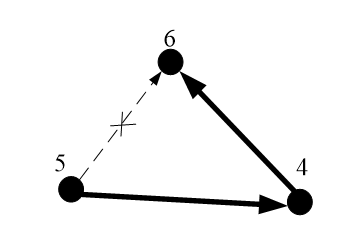Figure 6. Disconnection at edge (5, 6)

## 2.7.png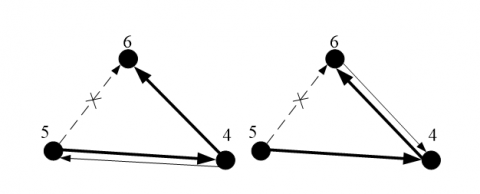Figure 7. Type B fault tolerance (Both edges (5, 4) and (4, 6) are added)

Hence, the detailed control laws can be expressed as:

$\overset{\centerdot }{\mathop{{{p}_{n-2}}}}\,={{k}_{n-2}}\frac{||{{p}_{n}}-{{p}_{n-2}}||-{{d}_{(n-2,n)\_o}}}{||{{p}_{n}}-{{p}_{n-2}}||}({{p}_{n}}-{{p}_{n-2}})$        (18)

$\overset{\centerdot }{\mathop{{{p}_{n-1}}}}\,={{k}_{n-1}}\frac{||{{p}_{n-2}}-{{p}_{n-1}}||-{{d}_{(n-1,n-2)\_o}}}{||{{p}_{n-2}}-{{p}_{n-1}}||}({{p}_{n-2}}-{{p}_{n-1}})$        (19)

$\overset{\centerdot }{\mathop{\delta {{p}_{n-2}}(t)}}\,={{k}_{n-2}}{{I}_{2}}{{R}_{e\_n-2}}{{R}_{((n-2)(n-1),00)}}\delta {{p}_{n-2}}(t)$      (20)

$R_{((n-2) n, 00)}=\left[\begin{array}{cccc}\bar{x}_{n-2}-\bar{x}_{n} & \bar{y}_{n-2}-\bar{y}_{n} & \bar{x}_{n}-\bar{x}_{n-2} & \bar{y}_{n}-\bar{y}_{n-2} \\ 0 & 0 & 0 & 0\end{array}\right]$      (21)

${{R}_{e\_n-2}}=\left[ \begin{array}{cc} {{\overline{x}}_{n}}-{{\overline{x}}_{n-2}} & {{\overline{y}}_{n}}-{{\overline{y}}_{n-2}} \\ -({{\overline{y}}_{n}}-{{\overline{y}}_{n-2}}) & -({{\overline{x}}_{n}}-{{\overline{x}}_{n-2}}) \end{array} \right]$       (22)

$\overset{\centerdot }{\mathop{\delta {{p}_{n-1}}(t)}}\,={{k}_{n-1}}{{I}_{2}}{{R}_{e\_n-1}}{{R}_{((n-1)(n-2),00)}}\delta {{p}_{n-1}}(t)$      (23)

$R_{((n-1)(n-2), 00)}=\left[\begin{array}{cccc}\bar{x}_{n-1}-\bar{x}_{n-2} & \bar{y}_{n-1}-\bar{y}_{n-2} & \bar{x}_{n-2}-\bar{x}_{n-1} & \bar{y}_{n-2}-\bar{y}_{n-1} \\ 0 & 0 & 0 & 0\end{array}\right]$      (24)

${{R}_{e\_n-1}}=\left[ \begin{array}{cc} {{\overline{x}}_{n-2}}-{{\overline{x}}_{n-1}} & {{\overline{y}}_{n-2}}-{{\overline{y}}_{n-1}} \\ -({{\overline{y}}_{n-2}}-{{\overline{y}}_{n-1}}) & -({{\overline{x}}_{n-2}}-{{\overline{x}}_{n-1}}) \end{array} \right]$        (25)

(C) Disconnection at edge (4, 5)

## 2.8.png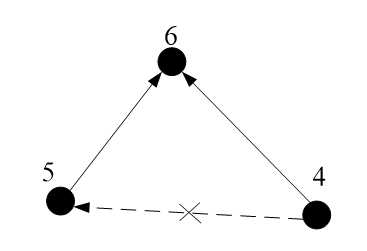Figure 8. Disconnection at edge (4, 5)

Finally, it is assumed that the edge between leaders 4 and 5 is broken (Figure 8). In this case, leaders 4 and 5 should change their orientation to receive information from leader 6. Hence, the detailed control laws can be expressed as:

$\overset{\centerdot }{\mathop{{{p}_{n-2}}}}\,={{k}_{n-2}}\frac{||{{p}_{n}}-{{p}_{n-2}}||-{{d}_{(n-2,n)\_o}}}{||{{p}_{n}}-{{p}_{n-2}}||}({{p}_{n}}-{{p}_{n-2}})$      (26)

$\overset{\centerdot }{\mathop{{{p}_{n-1}}}}\,={{k}_{n-1}}\frac{||{{p}_{n}}-{{p}_{n-1}}||-{{d}_{(n-1,n)\_o}}}{||{{p}_{n}}-{{p}_{n-1}}||}({{p}_{n}}-{{p}_{n-1}})$     (27)

$\overset{\centerdot }{\mathop{\delta {{p}_{n-2}}(t)}}\,={{k}_{n-2}}{{I}_{2}}{{R}_{e\_n-2}}{{R}_{((n-2)n,00)}}\delta {{p}_{n-2}}(t)$    (28)

$R_{((n-2)(n-2), 00)}=\left[\begin{array}{cccc}\bar{x}_{n-2}-\bar{x}_{n} & \bar{y}_{n-2}-\bar{y}_{n} & \bar{x}_{n}-\bar{x}_{n-2} & \bar{y}_{n}-\bar{y}_{n-2} \\ 0 & 0 & 0 & 0\end{array}\right]$      (29)

${{R}_{e\_n-2}}=\left[ \begin{array} {cc} {{\overline{x}}_{n}}-{{\overline{x}}_{n-2}} & {{\overline{y}}_{n}}-{{\overline{y}}_{n-2}} \\ -({{\overline{y}}_{n}}-{{\overline{y}}_{n-2}}) & -({{\overline{x}}_{n}}-{{\overline{x}}_{n-2}}) \end{array} \right]$      (30)

$\overset{\centerdot }{\mathop{\delta {{p}_{n-1}}(t)}}\,={{k}_{n-1}}{{I}_{2}}{{R}_{e\_n-1}}{{R}_{((n-1)n,00)}}\delta {{p}_{n-1}}(t)$    (31)

$R_{((n-1)n, 00)}=\left[\begin{array}{cccc}\bar{x}_{n-1}-\bar{x}_{n} & \bar{y}_{n-1}-\bar{y}_{n} & \bar{x}_{n}-\bar{x}_{n-1} & \bar{y}_{n}-\bar{y}_{n-1} \\ 0 & 0 & 0 & 0\end{array}\right]$      (32)

${{R}_{e\_n-1}}=\left[ \begin{array}{cc} {{\overline{x}}_{n}}-{{\overline{x}}_{n-1}} & {{\overline{y}}_{n}}-{{\overline{y}}_{n-1}} \\ -({{\overline{y}}_{n}}-{{\overline{y}}_{n-1}}) & -({{\overline{x}}_{n}}-{{\overline{x}}_{n-1}}) \end{array} \right]$      (33)

4.2.2 Control laws for the whole formation

The straightforward translation for the object is difficult to be discussed about the three co-leaders persistent formation. Without loss of generality, the general translation can be completed in two steps: x-axis translation and y-axis translation. Thus, the straightforward translation was replaced with x-axis and y-axis translations for analysis.

For $x$ -axis translation, if the leader that discovers the object is point $n,$ then the values of $\delta y_{n}, \delta x_{n}, \delta y_{n-1},$ and $\delta y_{n-2}$ are all zero, and the values of $\bar{y}_{n-2}-\bar{y}_{n-1}, \bar{y}_{n}-\bar{y}_{n-1},$ and $\bar{y}_{n-2}-\bar{y}_{n}$ are also zero. Then, we have:

$\left[\begin{array}{c}\delta \dot{x_{n-2}} \\ \delta \dot{y_{n-2}}\end{array}\right]=\left[\begin{array}{c} -k_{n-2}\left(\delta y_{n-2}-\delta y_{n-1} \\0 \right)\end{array}\right]$    (34)

$\left[\begin{array}{c}\delta \dot{x_{n-1}} \\ \delta \dot{y_{n-1}}\end{array}\right]=\left[\begin{array}{c} -k_{n-1}\left(\delta y_{n-1}-\delta y_{n-2} \\ 0 \right)\end{array}\right]$     (35)

\left[ \begin{align} & \overset{\centerdot }{\mathop{\delta {{x}_{n}}}}\, \\ & \overset{\centerdot }{\mathop{\delta {{y}_{n}}}}\, \\ \end{align} \right]=0      (36)

Likewise, for y-axis translation, we have:

$\left[\begin{array}{c}\delta \dot{x_{n-2}} \\ \delta \dot{y_{n-2}}\end{array}\right]=\left[\begin{array}{c}0 \\ -k_{n-2}\left(\delta y_{n-2}-\delta y_{n-1}\right)\end{array}\right]$    (37)

$\left[\begin{array}{c}\delta \dot{x_{n-1}} \\ \delta \dot{y_{n-1}}\end{array}\right]=\left[\begin{array}{c}0 \\ -k_{n-1}\left(\delta y_{n-1}-\delta y_{n-2}\right)\end{array}\right]$     (38)

\left[ \begin{align} & \overset{\centerdot }{\mathop{\delta {{x}_{n}}}}\, \\ & \overset{\centerdot }{\mathop{\delta {{y}_{n}}}}\, \\ \end{align} \right]=0      (39)

Obviously, x-axis translation is equivalent to y-axis translation. Thus, only x-axis translation is discussed here.

For the linear system, the new control laws can be expressed as:

$\left[\begin{array}{c}\dot{\delta x_{1}} \\ \dot{\delta y_{1}} \\ \dot{\delta x_{2}} \\ \delta \dot{ y_{2}} \\ \vdots \\ \delta \dot{x_{n-2}} \\ \delta \dot{x_{n-1}}\end{array}\right]$=AB$\left[\begin{array}{c}\delta x_{1} \\ \delta y_{1} \\ \delta x_{2} \\ \delta y_{2} \\ \vdots \\ \delta x_{n-2} \\ \delta x_{n-1}\end{array}\right]$      (40)

\begin{align} & A=\left[ \widehat{\alpha } \widehat{\beta } \widehat{\gamma } \right] \\ & B={{\left[ \widehat{{{K}_{\alpha }}}{{\widehat{{{R}_{e\_\alpha }}}}^{-1}}\widehat{{{R}_{\alpha }}} \widehat{{{K}_{\beta }}}{{\widehat{{{R}_{e\_\beta }}}}^{-1}}\widehat{{{R}_{\beta }}} \widehat{\gamma }\widehat{{{K}_{\gamma }}}{{\widehat{{{R}_{e\_\gamma }}}}^{-1}}\widehat{{{R}_{\gamma }}} \right]}^{T}} \\ \end{align}       (41)

$\text { where, } \widehat{K_{\alpha}}=\widehat{K_{\beta}}=\widehat{K_{\gamma}}=\left(\overset{ \begin{array}{c} i=1 \\ n-3 \end{array} }{\mathop{\oplus }}\, K_{i}\right) \oplus k_{n-2} \oplus k_{n-1}$

$A=\left\{\begin{array}{lll}{\left[\begin{array}{lll}1 & 0 & 0\end{array}\right] \text { When a form fault torellance }} \\ {\left[\begin{array}{lll}0 & 1 & 0\end{array}\right] \text { When b form fault torellance }} \\ {\left[\begin{array}{lll}0 & 0 & 1\end{array}\right] \text { When c form fault torellance }}\end{array}\right.$

Here, both $\widehat{R_{e}}$ and $\widehat{R}$ are $(2 n-4) \times(2 n-4), \widehat{R_{e}}$ is obtained by removing $(2 n-4),(2 n-2),(2 n-1)$ and $2 n$ rows and columns from $R_{e} ; \widehat{R}$ is obtained by removing $(2 \mathrm{n}-4),(2 \mathrm{n}-2)$ $(2 n-1), 2 n$ rows and $(2 n-3)$ column from $R$

Let $V^{\prime}$ be the set of followers, and $V_{f}^{\prime}$ be a subset of $V^{\prime} .$ Then, we have:

$\widehat{R_{\alpha}}=\left[\begin{array}{cccc}R\left(V^{\prime}\right) & & X \\ 0 & \bar{x}_{n-2}-\bar{x}_{n-1} & \bar{x}_{n-1}-\bar{x}_{n-2} & 0 \\ 0 & 0 & \bar{x}_{n-1}-\bar{x}_{n} & \bar{x}_{n}-\bar{x}_{n-1}\end{array}\right]$       (42)

$\widehat{{{R}_{e\_\alpha }}}=(\underset{i=1}{\overset{n-3}{\mathop{\oplus }}}\,{{E}_{i}})\oplus ({{\overline{x}}_{n-1}}-{{\overline{x}}_{n-2}})\oplus ({{\overline{x}}_{n}}-{{\overline{x}}_{n-1}} )$      (43)

$\widehat{R_{\beta}}=\left[\begin{array}{cccc}R\left(V^{\prime}\right) & & X \\ 0 & \bar{x}_{n-2}-\bar{x}_{n-1} & \bar{x}_{n-1}-\bar{x}_{n-2} & 0 \\ 0 & 0 & \bar{x}_{n-2}-\bar{x}_{n} & \bar{x}_{n}-\bar{x}_{n-2}\end{array}\right]$       (44)

$\widehat{{{R}_{e\_\beta }}}=(\underset{i=1}{\overset{n-3}{\mathop{\oplus }}}\,{{E}_{i}})\oplus ({{\overline{x}}_{n-2}}-{{\overline{x}}_{n-1}})\oplus ({{\overline{x}}_{n}}-{{\overline{x}}_{n-2}} )$    (45)

$\widehat{R_{\gamma}}=\left[\begin{array}{cccc}R\left(V^{\prime}\right) & & X \\ 0 & 0 & \bar{x}_{n-1}-\bar{x}_{n} & \bar{x}_{n}-\bar{x}_{n-1} \\ 0 & \bar{x}_{n-2}-\bar{x}_{n} & 0& \bar{x}_{n}-\bar{x}_{n-2} \end{array}\right]$        (46)

$\widehat{{{R}_{e\_\gamma }}}=(\underset{i=1}{\overset{n-3}{\mathop{\oplus }}}\,{{E}_{i}})\oplus ({{\overline{x}}_{n}}-{{\overline{x}}_{n-1}})\oplus ({{\overline{x}}_{n}}-{{\overline{x}}_{n-2}} )$     (47)

Suppose that the two outgoing edges from node i are ij and ik. Then, we have:

{{E}_{i}}=-\left[ \begin{align}{cc} {{x}_{i}}-{{x}_{j}} & {{y}_{i}}-{{y}_{j}} \\ {{x}_{i}}-{{x}_{k}} & {{y}_{i}}-{{y}_{k}} \\ \end{align} \right]     (48)

5. Conclusions

This paper explores deep into the emergency management of government data security based on the AI. Various AI techniques were created to ensure government data security, including intelligent government data security monitoring technology, ANN and fuzzy identification technology, and firewall security technology based fuzzy clustering. In the meantime, an AI-based security model was established on the novel concept of the GDRC. On this basis, the authors put forward an emergency management system for government data security, and verified its ability to safely control multiple datacenters through simulation of multi-agent formation. The future research will introduce more security factors to the proposed security model.

References

 Khan, Z., Pervez, Z., Ghafoor, A. (2014). Towards cloud based smart cities data security and privacy management. 2014 IEEE/ACM 7th International Conference on Utility and Cloud Computing, London, pp. 806-811. https://10.1109/UCC.2014.131

 Colin, T. (2012). Big data security. Network Security, 7: 5-8. https://10.1016/S1353-4858(12)70063-6

 Zhang, D. (2018). Big data security and privacy protection. Advances in Computer Science Research, 77: 275-278. https://doi.org/10.2991/icmcs-18.2018.56

 Ramachandran, M., Chang, V. (2016). Towards performance evaluation of cloud service providers for cloud data security. International Journal of Information Management, 36(4): 618-625. https://doi.org/10.1016/j.ijinfomgt.2016.03.005

 Gunasekaran, M., Chandu, T., Daphne, L., Revathi S. (2017). Big data security intelligence for healthcare industry 4.0. Cybersecurity for Industry 4.0, 7: 103-126. https://doi.org/10.1007/978-3-319-50660-9_5

 Syed, I.H.S., Vasilis, P., Ioannis, M. (2020). Government (Big) data ecosystem: definition, classification of actors, and their roles. International Journal of Computer and Information Engineering, 14(4): 102-114. https://doi.org/10.5281/zenodo

 Edoardo, C., Simone, C., Marco, G., Mauro, I., Davide, M., Pietro, P. (2019). Modeling and evaluating a complex edge computing based systems: An emergency management support system case study. Internet of Things, 6: 156-168. https://doi.org/10.1016/j.iot.2019.100054

 Puthal, D., Nepal, S., Ranjan R., Chen, J. (2016). A secure big data stream analytics framework for disaster management on the cloud. 2016 IEEE 18th International Conference on High Performance Computing and Communications; IEEE 14th International Conference on Smart City; IEEE 2nd International Conference on Data Science and Systems (HPCC/SmartCity/DSS), Sydney, NSW, pp. 1218-1225 https://doi.org/10.1109/HPCC-SmartCity-DSS.2016.0170

 Carminati, B., Ferrari, E., Guglielmi, M. (2016). Detection of unspecified emergencies for controlled information sharing. IEEE Transactions on Dependable and Secure Computing, 13(6): https://doi.org/630-643.10.1109/TDSC.2015.2427846

 Chen, C., Li, C., Liu, S., Wu T., Pan, J. (2017). A provable secure private data delegation scheme for mountaineering events in emergency system. IEEE Access, 5: 3410-3422. https://doi.org/10.1109/ACCESS.2017.2675163

 Jackson, D., Hayes, P. (2016). Ensuring security of data and information flow in emergency response decision support. 2016 11th International Conference on Availability, Reliability and Security (ARES), Salzburg, pp. 792-797. https://doi.org/10.1109/ARES.2016.49

 Cao, H., Bai, Y., Chen, J., Fang, H. (2013). Control of 2D minimally persistent formations with three co-leaders in a cycle. International Journal of Advanced Robotic Systems, 210(21): 1-7. https://doi.org/10.5772/54494

 Cao, H., Bai, Y., Liu, H. (2012). Distributed rigid formation control algorithm for multi-agent systems. Kybernetes, 41(10): 1650-1661. https://doi.org/10.1108/03684921211276819

 Olfati-Saber, R., Murray, R. (2002). Distributed cooperative control of multiple vehicle formations using structural potential functions. Proc. of 15th IFAC World Congress, 35(1): 495-500. https://doi.org/10.3182/20020721-6-es-1901.00244

 Jiang, L., Zhang, R. (2012). Stable formation control of multi-robot system with communication delay. International Journal of Advanced Robotic Systems, 9(1): 1-10. https://doi.org/10.5772/45603

 Tanner, H., Jadbabaie, A., Pappas, G.J. (2003). Stable flocking of mobile agents, part I: fixed topology. 42nd IEEE International Conference on Decision and Control (IEEE Cat. No.03CH37475), Maui, HI, pp. 2010-2015. https://doi.org/10.1109/CDC.2003.1272910

 Saulnier, K., Saldaña, D., Prorok, A., Pappas, G.J., Kumar, V. (2017). Resilient flocking for mobile robot teams. IEEE Robotics and Automation Letters, 2(2): 1039-1046. https://doi.org/10.1109/LRA.2017.2655142

 Ramazani, S., Selmic, R., de Queiroz, M. (2017). Rigidity-based multiagent layered formation control. IEEE Transactions on Cybernetics, 47(8): 1902-1913. https://doi.org/10.1109/TCYB.2016.2568164

 Krick, L., Broucke, M.E., Francis, B.A. (2009). Stabilisation of infinitesimally rigid formations of multi-robot networks. International Journal of Control, 82(3): 423-439. https://doi.org/10.1109/CDC.2008.4738760

 Asimow, L., Roth, B. (1978). Rigidity of graphs, II. Journal of Mathematical Analysis and Applications, 68(1): 171-190. https://doi.org/10.1016/0022-247x(79)90108-2

 Yu, C., Anderson, B.D.O., Dasgupta, S. (2009). Control of minimally persistent formations in the plane. Siam Journal on Control and Optimization, 48(1): 206-233. https://doi.org/10.1137/060678592